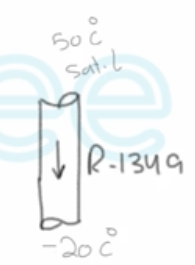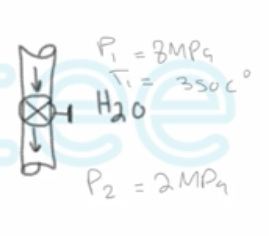Need Help?

Subscribe to Thermodynamics

###### \${selected_topic_name}
• Notes

$\text { An adiabatic capillary tube is used in some refrigeration systems to drop the pressure of the refrigerant }$

$\begin{array}{l}{\text { from the condenser level to the evaporator level. The } R-134 \text { a enters the capilary tube as a saturated liquid }} \\ {\text { at } 50^{\circ} \mathrm{C}, \text { and leaves at }-20^{\circ} \mathrm{C} \text { . Determine the quality of the refrigerant at the evaporator. }}\end{array}$$\dot{E}_{i n}=\dot{E}_{\text {out }}$

$\dot mh_{1}=\dot m h _{2}$

$h_{1}=h_{2}$

$A-11 \longrightarrow @ \ T_{1}=50 c^{\circ} \longrightarrow h_1=h_f=123.49 kJ/kg$

$\left.\begin{array}{l}{T_{2}=-20 c^{\circ}} \\ {h_{2}=h_{1}=123.4 9 k{J} /k g}\end{array}\right\} \rightarrow X_{2}=\frac{h_2-h_f}{h_{fg}}$

$=\frac{123.49-25.49}{212.91}$

$=0.046$

$\begin{array}{l}{\text { A well-insulated valve is used to throttle steam from } 8 \text { MPa and } 350^{\circ} \mathrm{C} \text { to } 2 \mathrm{MPa} \text { . }} \\ {\text { Determine the final temperature of the steam }}\end{array}$$\left.\begin{array}{l}{P_{1}=8 \mathrm{MPa}} \\ {T_{1}=350^{\circ} \mathrm{C}}\end{array}\right] \rightarrow \mathrm{A}-6$

$\rightarrow h_{1}=2988.1 \mathrm{kJ} / \mathrm{kg}$

$\dot m_{1}=\dot m_{2}=\dot m$

$\dot E_{\text {in }}=\dot{E}_{\text {out }}$

$\dot m h_1=\dot{m} h_{2} \quad \rightarrow h_{1}=h_{2}=2988.1 kJ/kg$

$\left.\begin{array}{l}{P_{2}=2 M Pa} \\ {\left(h_{2}=h_{1}=2988 \cdot 1\right)}\end{array}\right] \rightarrow T_{2}=285 {c^{\circ}}$